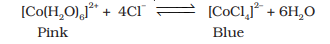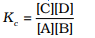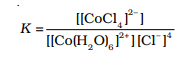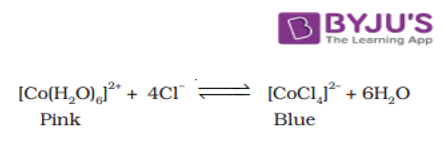Checkout JEE MAINS 2022 Question Paper Analysis : Checkout JEE MAINS 2022 Question Paper Analysis :

# Study The Shift In Equilibrium Between [Co(H2O)6]2+ And Chloride Ions By Changing The Concentration Of Either Of The Ions

Let us study the equilibrium reaction between [Co(H2O)6]2+ and chloride ions through the change in the concentration of the ions. Following displacement takes place in the chemical reaction between [Co(H2O)6]2+ and chloride.The expression for the equilibrium constant for the general reaction

$$\begin{array}{l}A + B \rightarrow C + D\end{array}$$
is given as:The expression for the equilibrium constant for the reaction between [Co(H2O)6]2+ and chloride ions is given as:This reaction takes place in an aqueous medium where the concentration of H2O remains constant.

At equilibrium either [Co(H2O)6] 2+ ion or Cl ions concentration is increased, and this would result in an increase in [CoCl4] 2– ion concentration thus, maintaining the value of K as constant. There is a shift in the equilibrium in the forward direction along with the change in colour.

## Aim:

To understand the process of shift in equilibrium between [Co(H2O)6] 2+ and chloride ions by changing the concentration of either of the ions.

## Materials Required:

60 mL Acetone/alcohol, 30 mL Concentrated hydrochloric acid, Cobalt chloride 0.6000 g, 100 mL Conical flask, 100 mL Beakers – 3, 3 Burettes, 6 Test tubes, Test tube stand, Glass rod

## Procedure:

1. In a 100 mL conical flask, take 60 mL of acetone and dissolve 0.6000 g of CoCl2 to get a blue solution.
2. Make sure you have 5 test tubes of the same volume. Mark them as A, B, C, D, and E.
3. Now add 3.0 mL of cobalt chloride solution into each of the test tubes.
4. Also add 1.0 mL, 0.8 mL, 0.6 mL, 0.4 mL and 0.2 mL of acetone into the test tubes A, B, C, D, and E.
5. Add 0.2 mL, 0.4 mL, 0.6 mL and 0.8 mL of water to test tubes B, C, D and E respectively, so that the total volume of solution in each of the test tubes is 4.0 mL.
6. As the quantity of water increases, the mixture turns from blue to pink. Note the changes.
7. Take 10 mL cobalt chloride solution in acetone prepared above and add 5 mL distilled water to it. A solution of pink colour will be obtained.
8. Take 1.5 mL of pink solution in five different test tubes labelled as A′ B′, C′, D′, and E′.
9. Add 2.0 mL, 1.5 mL, 1.0 mL and 0.5 mL of water to the test tubes labelled from A′ to D′ and 0.5 mL, 1.0 mL, 1.5 mL, 2.0 mL and 2.5 mL concentrated HCl respectively in the test tubes A′ to E′ so that total volume of solution in the test tubes is 4 mL.
10. The colour gradually changes from pink to light blue with increasing amounts of hydrochloric acid. Record observations in the tables given below.

## Precautions to be taken during the experiment:

• Use only distilled water.
• Use a burette or graduated pipette for adding water or solutions.
• Handle Acetone and alcohol with care, since it is flammable

## Observation

Study of equilibrium shift when adding water

 Test Tube The volume of acetone added The volume of CoCl2 solution added The volume of water added Colour of mixture A 1.0 3.0 0.0 B 0.8 3.0 0.2 C 0.6 3.0 0.4 D 0.4 3.0 0.6 E 0.2 3.0 0.8

Study of Equilibrium shift on adding Cl ions

 Test Tube The volume of concentrated HCl added The volume of aqua complex solution added The volume of water added Colour of mixture A 0.5 1.5 2.0 B 1.0 1.5 1.5 C 1.5 1.5 1.0 D 2.0 1.5 0.5 E 2.5 1.5 0.0

## Viva Voce:

1. What happens when the temperature of the reaction mixture is increased at equilibrium?

Ans: It reduces the effect of change in the reaction.

2. Is it correct to replace the aqueous solution of sodium chloride with concentrated HCl?

Ans: No, since adding concentrated hydrochloric acid raises the chloride ion concentration, causing the equilibrium to move to the right.

3. Why is it necessary to keep the total volume of the solution equivalent in each test tube?

Ans: To study the shift in the equilibrium and to know the exact measurement of the reactants added, the test tubes are filled with the same quantity of solution.

4. Give an example of ligand displacement reaction?

Ans:Test Your Knowledge On Process Of The Shift In Equilibrium Experiment!